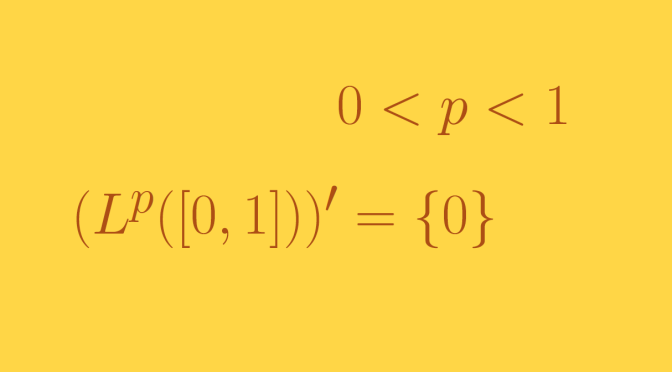A topological vector space with no non trivial continuous linear form

We consider here the $$L^p$$- spaces of real functions defined on $$[0,1]$$ for which the $$p$$-th power of the absolute value is Lebesgue integrable. We focus on the case $$0 < p < 1$$. We'll prove that those $$L^p$$-spaces are topological vector spaces on which there exists no continuous non-trivial linear forms (i.e. not vanishing identically).

The elements of $$L^p$$ are those Lebesgue measurable functions $$f$$ on $$[0, 1]$$ for which:
$\Delta(f)=\int_0^1 \vert f(t) \vert^p dt < \infty$ Since $$0 < p < 1$$, the inequality $(a+b)^p \le a^p + b^p$ holds for $$a \ge 0$$ and $$b \ge 0$$. This gives $\Delta(f+g) \le \Delta(f) + \Delta(g)$ so that $d(f,g) = \Delta(f-g)$ is an invariant metric on $$L^p$$. This metric induces a topology on the space $$L^p$$. Equipped with this topology, $$L^p$$ is a topological vector space as the maps $$(x,y) \mapsto x+y$$ from $$L^p \times L^p$$ into $$L^p$$ and $$(\lambda,x) \mapsto \lambda x$$ from $$\mathbb{R} \times L^p$$ into $$L^p$$ are continuous.

$$L^p$$ ($$0 < p < 1$$) contains no convex open sets other than $$\emptyset$$ and $$L^p$$

Suppose that $$V$$ is a non-empty convex open set in $$L^p$$. We can assume that $$0 \in V$$ possibly using a translation. As $$V$$ is open, one can find $$r > 0$$ with $$B_r = \{f \in L^p \text{ : } \Delta(f) < r\} \subset V$$. Pick $$f \in L^p$$. Since $$p < 1$$, there is a positive integer $$n$$ such that $$n^{p-1} \Delta(f) < r$$. By the continuity of the indefinite integral of $$\vert f \vert^p$$, there are points $$0 = x_0 < x_1 < \dots < x_n=1$$ such that $\int_{x_{i-1}}^{x_i} \vert f(t) \vert^p dt = \frac{\Delta(f)}{n} \text{ for } 1 \le i \le n$ For $$1 \le i \le n$$ define $$g_i(t)=nf(t)$$ for $$x_{i-1} < t \le x_i$$, $$g_i(t)=0$$ otherwise. We have: $\Delta(g_i)=\int_{x_{i-1}}^{x_i} \vert nf(t) \vert^p dt=n^{p-1} \Delta(f) < r$ Hence $$g_i \in B_r$$ and $$g_i \in V$$ as $$B_r \subset V$$. Since $$V$$ is supposed to be convex and $$f=\frac{1}{n}(g_1+\dots+g_n)$$ it follows that $$f \in V$$. Therefore $$V = L^p$$.

There exists no continuous non-trivial linear form on $$L^p$$

Suppose $$\Lambda: \ L^p \to \mathbb{R}$$ is a continuous linear form. The inverse image of the open convex subset $$(-1,1) \subset \mathbb{R}$$ under $$\Lambda$$ is convex as $$\Lambda$$ is linear and open as $$\Lambda$$ is continuous. According to previous paragraph, if $$\Lambda$$ is not vanishing identically, $$\Lambda^{-1}((-1,1)) = L^p$$ which is not possible. Therefore $$0$$ is the only continuous linear form.